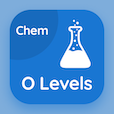Cambridge Online Courses (GCSE)

O Level Chemistry Quizzes

O Level Chemistry Quiz PDF - Complete

# Chemical Symbols Multiple Choice Questions p. 38

Study Chemical Symbols multiple choice questions and answers, chemical symbols quiz answers PDF 38 to study O Level Chemistry course online. Chemical Formulae and Equations MCQ trivia questions, chemical symbols Multiple Choice Questions (MCQ) for online college degrees. "Chemical Symbols MCQ" PDF eBook: periodic table: o level chemistry, chemical symbols test prep for GRE practice test.

"The formula of aluminum sulfate indicates that for" MCQ PDF: each ion of al, three ions of sulphate are required, each ion of al, two ions of sulphate are required, two ions of al, 3 ions of sulphate are required, and two ions of al, three ions of sulphate are required for online colleges for science. Learn chemical formulae and equations questions and answers to improve problem solving skills for best ACT prep courses online.

## Chemical Symbols Questions and Answers MCQs

MCQ: The formula of aluminum sulfate indicates that for

each ion of Al, two ions of sulphate are required
each ion of Al, three ions of sulphate are required
two ions of Al, 3 ions of sulphate are required
two ions of Al, three ions of sulphate are required

MCQ: Elements with full outermost shells are

metals
non-metals
inert gases
neutral oxides

MCQ: Insect bites and stinging nettles contain

formic acid
tartaric acid
oxalic acid
malic acid

MCQ: All of these ions have a valency of +2 except

Pb
Ca
Zn
NH4

MCQ: A water molecule will always contain

11% of oxygen and 89% of hydrogen
18% of oxygen and 88% of hydrogen
11.11% of oxygen and 88.89% of hydrogen
15% of oxygen

### More Quizzes from O Level Chemistry Course